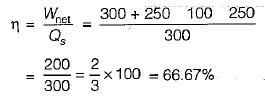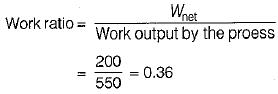Courses

# Test: First Law of Thermodynamics - 1

## 10 Questions MCQ Test Topicwise Question Bank for Mechanical Engineering | Test: First Law of Thermodynamics - 1

Description
This mock test of Test: First Law of Thermodynamics - 1 for Mechanical Engineering helps you for every Mechanical Engineering entrance exam. This contains 10 Multiple Choice Questions for Mechanical Engineering Test: First Law of Thermodynamics - 1 (mcq) to study with solutions a complete question bank. The solved questions answers in this Test: First Law of Thermodynamics - 1 quiz give you a good mix of easy questions and tough questions. Mechanical Engineering students definitely take this Test: First Law of Thermodynamics - 1 exercise for a better result in the exam. You can find other Test: First Law of Thermodynamics - 1 extra questions, long questions & short questions for Mechanical Engineering on EduRev as well by searching above.
QUESTION: 1

### The first law of thermodynamics is the law of

Solution:

The First Law of Thermodynamics is basically based on the Law of Conservation of Energy.

QUESTION: 2

### A PMM1 is

Solution:

There can be no machine which would continuously supply mechanical work without some other form of energy disappearing simultaneously. It is fictitious machine.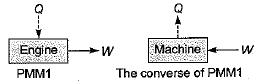QUESTION: 3

### In a cyclic process, heat transfer are +15.7 kJ, -26.2 kJ, -4.86 kJ and +31.5 kJ. What is the network for this cyclic process?

Solution: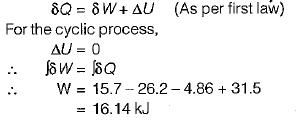QUESTION: 4

A stationary mass of gas is compressed without friction from an initial state of 0.3 m3 and 0.1 MPa to a final state of 0.15 m3 and 0.1 MPa, the pressure remaining constant during the process. There is a transfer of 40 kJ of heat from the gas during the process. What is the change in internal energy of the gas?

Solution: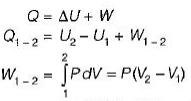= 0.1 χ 106 (0.15-0.3)
= -15Kj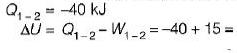-25Kj

QUESTION: 5

An engine is tested by means of a water brake at 1000 rpm. The measured torque-of the engine is 10000 Nm and the water consumption of the brake is 0.5 m3/s, its inlet temperature being 25°C. Assuming that the whole of the engine power is ultimately transferred into heat which is absorbed by the cooling water, what is the water temperature at exit?

Solution:

Engine power,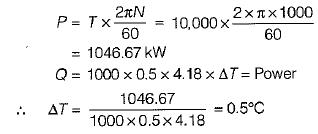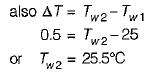QUESTION: 6

What reaction takes place during photosynthesis?

Solution:

Photosynthesis takes place by absorbing heat and energy from the surroundings. Since, endothermic reaction is a reaction in which the system absorbs heat from its surroundings, the reaction that takes place during photosynthesis is an endothermic reaction.

QUESTION: 7

What is the temperature rise after 5 minutes in the below volume?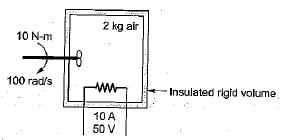Solution:

Energy in = Energy out

Energy in = 10 x 100 x 5 x 60 + 10 x 50 x 5 x 60 = 450 kJ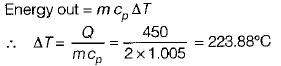QUESTION: 8

Energy is added to 5 kg of air with a paddle wheel so that ΔT = 100°C while P = const. in an insulated container. The paddle-wheel work is

Solution:

–W = mΔh

= 5 × CpΔT

= 5 × 1.0 × 100

= 500 kJ

QUESTION: 9

An ideal gas of mass m at state 1 expands to state 2 via three paths. If QA, QB and Qc represent the heat absorbed by the gas along three paths, then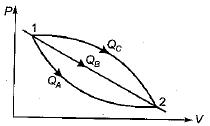Solution: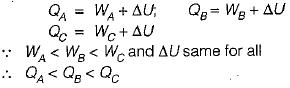QUESTION: 10

The values of heat and work transfer for the flow processes of a thermodynamic cycle are given below: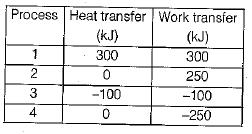The thermal efficiency and work ratio for the cycle will be respectively

Solution: